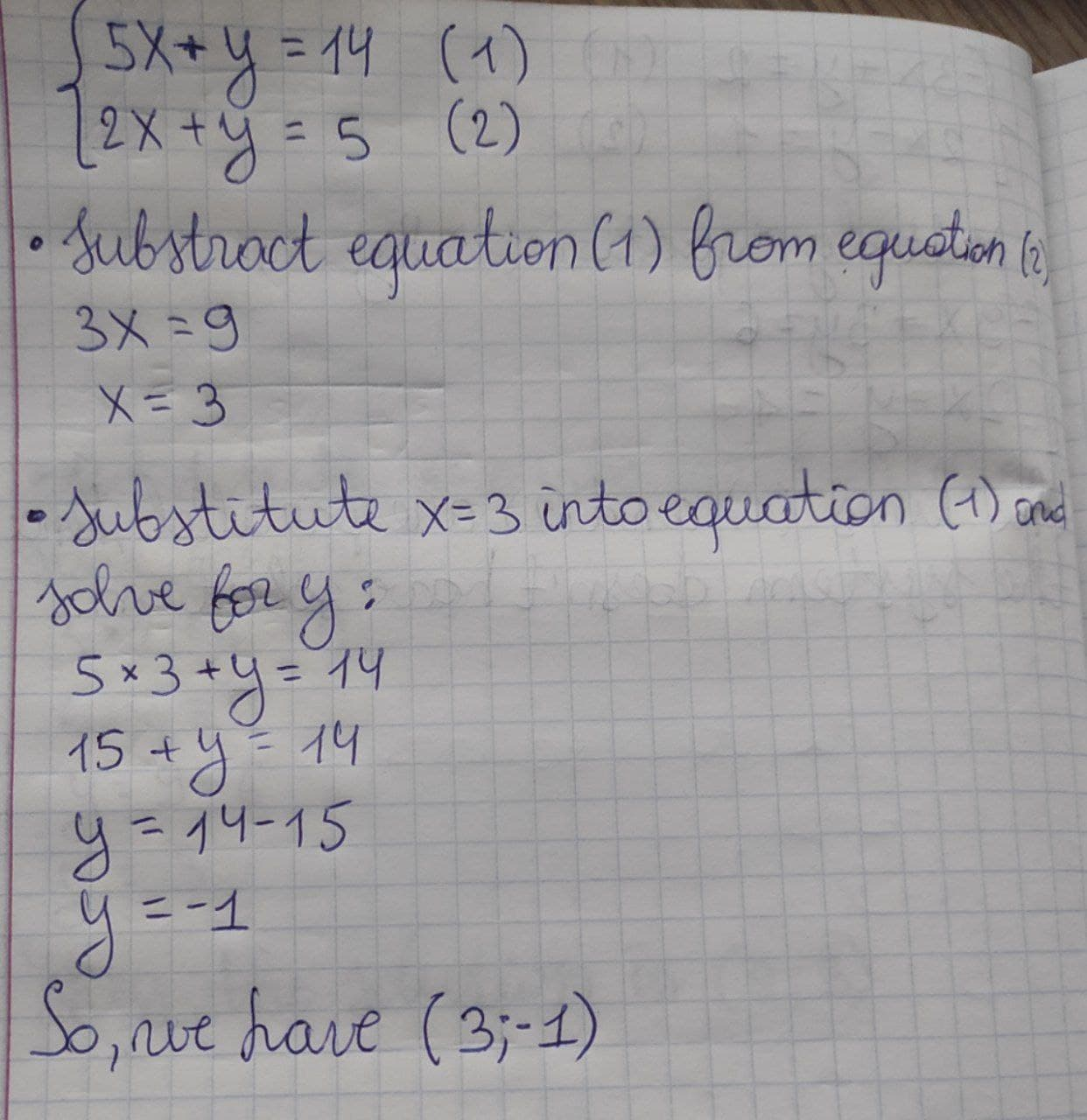Ask question

# Please, solve the system of equations: {(5x,+,y,=,14),(2x,+,y,=,5):}# Please, solve the system of equations: {(5x,+,y,=,14),(2x,+,y,=,5):}

Question
Systems of equationsasked 2021-01-19
Please, solve the system of equations: {(5x,+,y,=,14),(2x,+,y,=,5):}

## Answers (1)2021-01-20

{(5x,+,y,=,14),(2x,+,y,=,5):} Subtract equation (1) from equation (2): 3x = 9 Find the x: x = 9/3 x = 3 Substitute x =3 into equation (1) and solve for y: 5 xx 3 + y = 14 15 + y = 14 y = 14 – 15 y = -1 So we have (3,-1)### Relevant Questionsasked 2021-02-02

Solve the system of equations (Use matrices.):
$$x-2y+z = 16$$,
$$2x-y-z = 14$$,
$$3x+5y-4z =-10$$asked 2021-04-26
Solve the system of equations.
y=2x-5
3x+2y=18asked 2021-02-20
What is the solution of the system of equations?
y = 2x - 3
5x + y = 11
A (2, 1)
B (1, 2)
C (3, -4)
D (1, -1)asked 2020-11-22
Solve: {(5x,-,4y,=,-5),(3x,+,y,=,14):}asked 2020-10-23

Determine if (1,3) is a solution to the given system of linear equations.

$$5x+y=8$$

$$x+2y=5$$asked 2021-02-10
Solve the system: {(-2x,+,y,=,5),(-6x,+,3y,=,21):}asked 2021-03-22
Solve the system of equations x+y=-1 and 5x-7y=79 by combining the equations.asked 2021-02-19
Solve the system of equations. {(x,+,4y,=,-2),(-2x,+12y,=,9):}asked 2021-03-12

Determine whether the ordered pair is a solution to the given system of linear equations.
(5,3)
$$x-y=2$$
$$x+y=8$$asked 2021-03-23
Solve the system of equations.
2x-y=6
$$\displaystyle{x}^{{{2}}}+{y}={9}$$
...